7:47 PM
Mass and Energy Michael Fowler, University of Virginia 3/1/2008

# Mass and Energy

Michael FowlerUniversity of Virginia   3/1/2008

### Rest Energy

The fact that feeding energy into a body increases its mass suggests that the mass m0 of a body at rest, multiplied by c2, can be considered as a quantity of energy.  The truth of this is best seen in interactions between elementary particles.  For example, there is a particle called a positron which is exactly like an electron except that it has positive charge.  If a positron and an electron collide at low speed (so there is very little kinetic energy) they both disappear in a flash of electromagnetic radiation.  This can be detected and its energy measured.  It turns out to be 2m0c2 where m0 is the mass of the electron (and the positron).

Thus particles can “vaporize” into pure energy, that is, electromagnetic radiation.  The energy m0c2 of a particle at rest is called its “rest energy”.  Note, however, that an electron can only be vaporized by meeting with a positron, and there are very few positrons around normally, for obvious reasons-they just don’t get far.  (Although occasionally it has been suggested that some galaxies may be antimatter!)

### Einstein’s Box

An amusing “experiment” on the equivalence of mass and energy is the following: consider a closed box with a flashlight at one end and light-absorbing material at the other end.  Imagine the box to be far out in space away from gravitational fields or any disturbances.  Suppose the light flashes once, the flash travels down the box and is absorbed at the other end.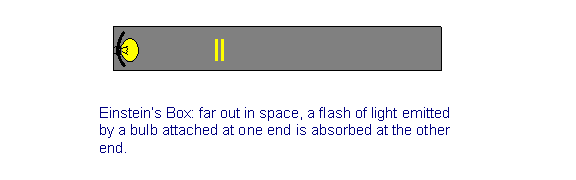Now it is known from Maxwell’s theory of electromagnetic waves that a flash of light carrying energy E also carries momentum p = E/c. Thus, as the flash leaves the bulb and goes down the tube, the box recoils, like a gun, to conserve overall momentum.  Suppose the whole apparatus has mass M and recoils at velocity v.  Of course, v << c.

Then from conservation of momentum in the frame in which the box was initially at rest: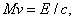the recoil momentum of the box equals (minus) the momentum of the flash emitted.

After a time t = L/c the light hits the far end of the tube, is absorbed, and the whole thing comes to rest again.  (We are assuming that the distance moved by the box is tiny compared to its length.)

How far did the box move?  It moved at speed v for time t, so it moved distance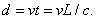From the conservation of momentum equation above, we see that v = E/Mc, so the distance d the box moved over is: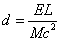Now, the important thing is that there are no external forces acting on this system, so the center of mass cannot have moved!

The only way this makes sense is to say that to counterbalance the mass M moving d backwards, the light energy must have transferred a small mass m, say, the length L of the tube so that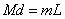and balance is maintained. From our formula for above, we can figure out the necessary value of m,so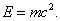We have therefore established that transfer of energy implies transfer of the equivalent mass.  Our only assumptions here are that the center of mass of an isolated system, initially at rest, remains at rest if no external forces act, and that electromagnetic radiation carries momentum E/c, as predicted by Maxwell’s equations and experimentally established.

But how is this mass transfer physically realized?  Is the front end of the tube really heavier after it absorbs the light?  The answer is yes, because it’s a bit hotter, which means its atoms are vibrating slightly faster—and faster moving objects have higher mass. (And there’s another contribution we’re about to discuss.)

### Mass and Potential Energy

Suppose now at the far end of the tube we have a hydrogen atom at rest.  As we shall see later, this atom is essentially a proton having an electron bound to it by electrostatic attraction.  It is known that a flash of light with total energy 13.6eV is just enough to tear the electron away, so in the end the proton and electron are at rest far away from each other.  The energy of the light was used up dragging the proton and electron apart—that is, it went into potential energy.  (It should be mentioned that the electron also loses kinetic energy in this process, 13.6 ev is the net energy required to break up the atom.)  Now, the light is absorbed by this process, so from our argument above the right hand end of the tube must become heavier.  That is to say, a proton at rest plus a (distant) electron at rest weigh more than a hydrogen atom by E/c2, with E equal to 13.6eV.  Thus, Einstein’s box forces us to conclude that increased potential energy in a system also entails the appropriate increase in mass.

It is interesting to consider the hydrogen atom dissociation in reverse—if a slow moving electron encounters an isolated proton, they may combine to form a hydrogen atom, emitting 13.6eV of electromagnetic radiation energy as they do so.  Clearly, then, the hydrogen atom remaining has that much less energy than the initial proton + electron.  The actual mass difference for hydrogen atoms is about one part in 108.  This is typical of the energy radiated away in a violent chemical reaction—in fact, since most atoms are an order of magnitude or more heavier than hydrogen, a part in 109 or 1010 is more usual.  However, things are very different in nuclear physics, where the forces are stronger so the binding is tighter.  We shall discuss this later, but briefly mention an example:  a hydrogen nucleus can combine with a lithium nucleus to give two helium nuclei, and the mass shed is 1/500 of the original.  This reaction has been observed, and all the masses involved are measurable.  The actual energy emitted is 17 MeV.  This is the type of reaction that occurs in hydrogen bombs.  Notice that the energy released is at least a million times more than the most violent chemical reaction.

As a final example, let’s make a ballpark estimate of the change in mass of a million tons of TNT on exploding.  The TNT molecule is about a hundred times heavier than the hydrogen atom, and gives off a few eV on burning.  So the change in weight is of order 10-10 x106 tons, about a hundred grams.  In a hydrogen bomb, this same mass to energy conversion would take about fifty kilograms of fuel.

### Footnote: Einstein’s Box is a Fake

Although Einstein’s box argument is easy to understand, and gives the correct result, it is based on a physical fiction—the rigid box.  If we had a rigid box, or even a rigid stick, all our clock synchronization problems would be over—we could start clocks at the two ends of the stick simultaneously by nudging the stick from one end, and, since it’s a rigid stick, the other end would move instantaneously.  Actually there are no such materials.  All materials are held together by electromagnetic forces, and pushing one end causes a wave of compression to travel down the stick.  The electrical forces between atoms adjust at the speed of light, but the overall wave travels far more slowly because each atom in the chain must accelerate for a while before it moves sufficiently to affect the next one measurably.  So the light pulse will reach the other end of the box before it has begun to move!  Nevertheless, the wobbling elastic box does have a net recoil momentum, which it does lose when the light hits the far end.  So the basic point is still valid.  French gives a legitimate derivation, replacing the box by its two (disconnected) ends, and finding the center of mass of this complete system, which of course remains at rest throughout the process

Category: Education | Views: 445 | Added by: farrel | | Rating: 0.0/0